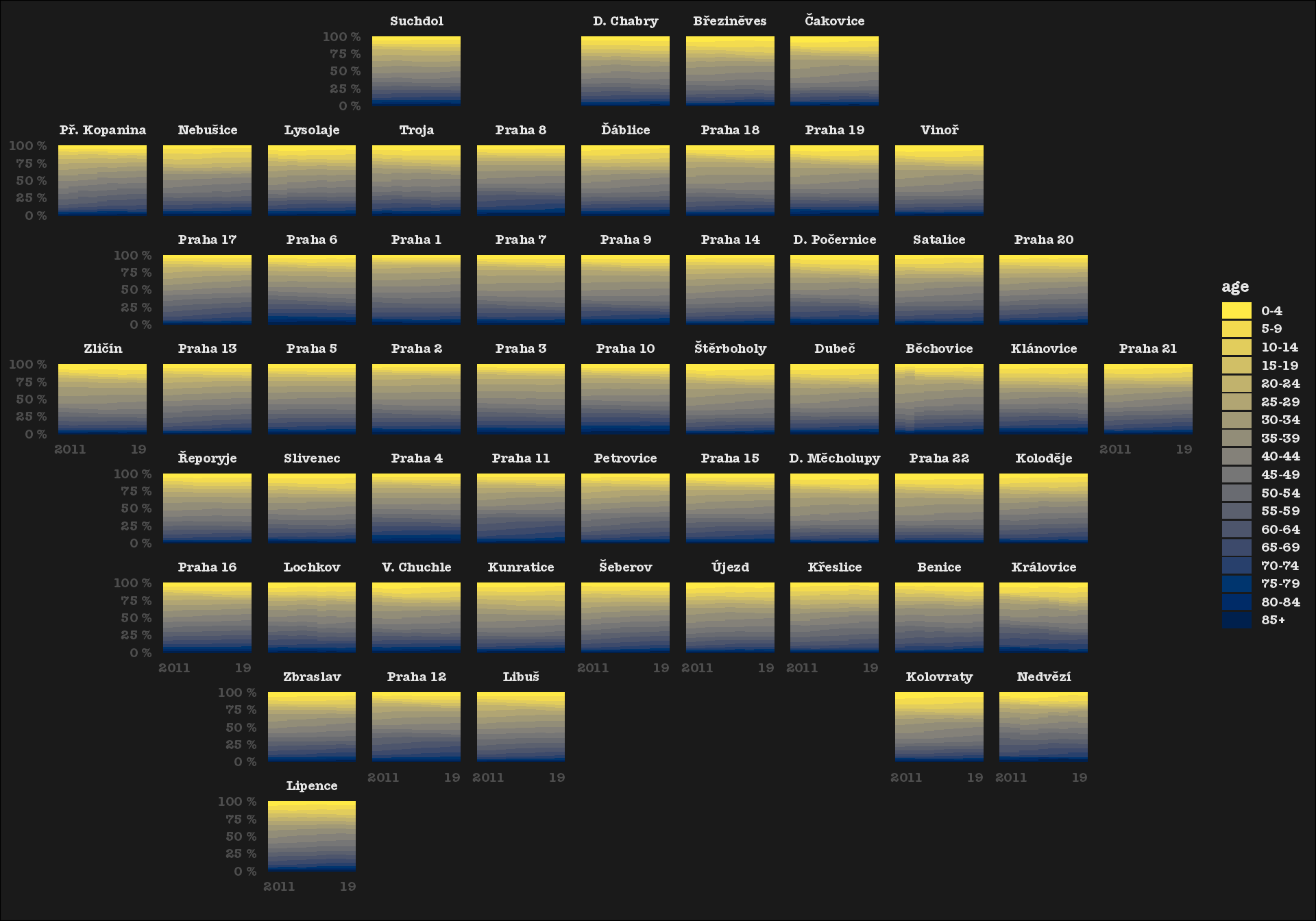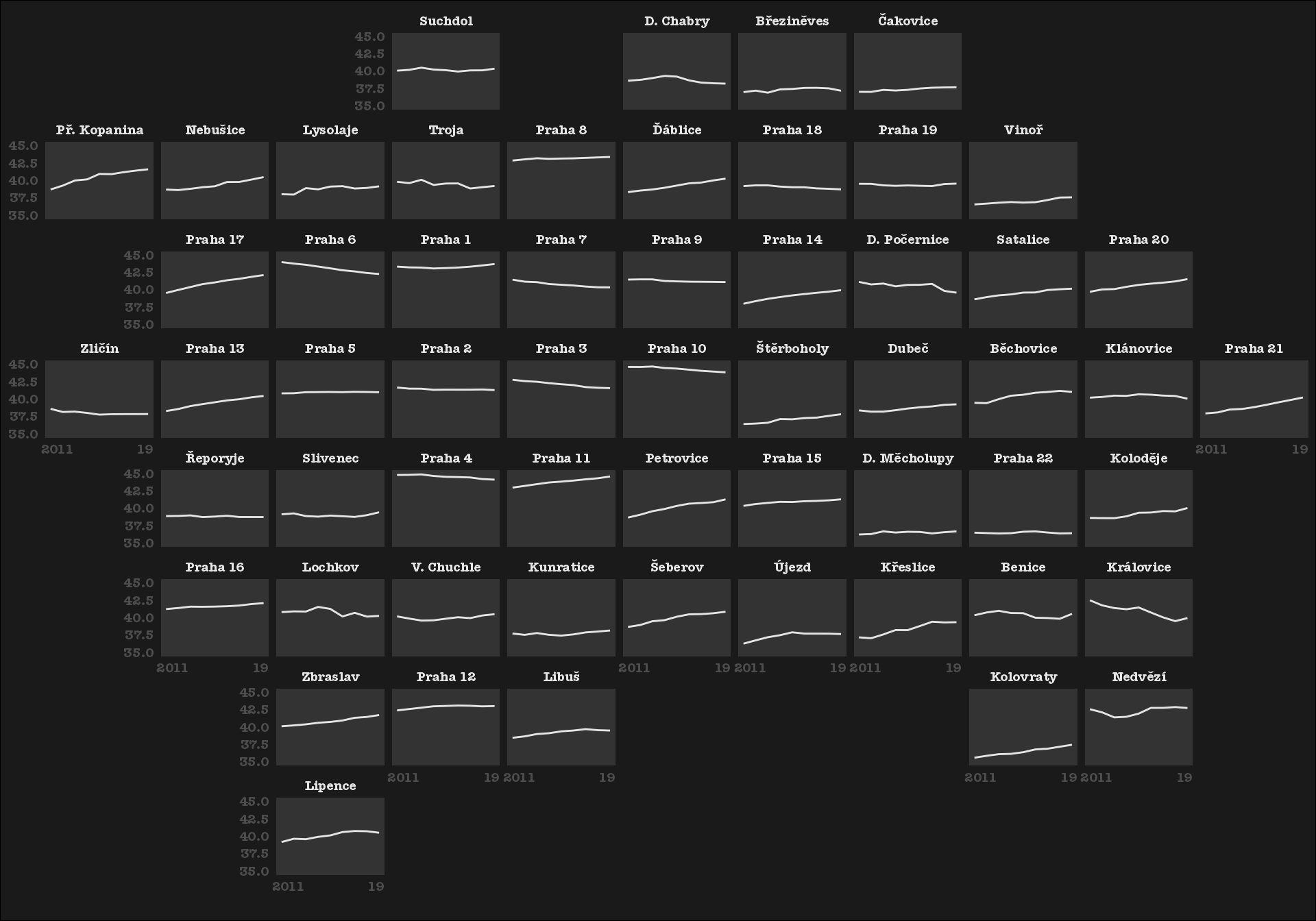library(pragr)
library(CzechData)
#> Linking to GEOS 3.8.1, GDAL 3.1.4, PROJ 6.3.1
library(geofacet)
library(ggplot2)
library(dplyr)
#>
#> Attaching package: 'dplyr'
#> The following objects are masked from 'package:stats':
#>
#>     filter, lag
#> The following objects are masked from 'package:base':
#>
#>     intersect, setdiff, setequal, union
library(sf)

mcds <- phamc0 %>%
mutate(area = st_area(geometry) %>% units::drop_units(),
h = 1) %>%
st_set_geometry(NULL) %>%
select(code = kod, area, h)
d_age <- district_age_structure %>%
rename(code = KOD_ZUJ) %>%
filter(age != "Total")

ggplot(d_age) +
geom_col(aes(x = year, y = count, fill = age), position = "fill", width = 1) +
facet_geo(~ code, grid = district_geofacet %>%
left_join(district_names %>% select(code,
name_label = name_short)),
label = "name_label") +
theme_minimal(base_family = "Unit Pro", base_size = 9) +
theme(text = element_text(colour = "grey90", face = "bold"),
plot.background = element_rect(fill = "grey10"),
panel.grid = element_blank(),
axis.ticks = element_blank(),legend.key.height = unit(10, "pt"),
panel.background = element_rect(fill = "grey10", colour = NA),
strip.text = element_text(colour = "grey90")) +
scale_x_continuous(breaks = c(min(d_age$year), max(d_age$year)),
labels = c("    2011", "19  "),
name = "") +
scale_fill_viridis_d(option = "E", direction = -1) +
scale_y_continuous(labels = scales::label_percent(suffix = " %"), name = NULL)
#> Joining, by = "code"
#> Note: You provided a user-specified grid. If this is a generally-useful
#>   grid, please consider submitting it to become a part of the geofacet
#>   package. You can do this easily by calling:
#>   grid_submit(__grid_df_name__)d_median <- district_age_median %>%
filter(sex == "Total") %>%
rename(code = KOD_ZUJ)

ggplot(d_median) +
geom_line(aes(x = year, y = median_age), colour = "grey90") +
facet_geo(~ code, grid = district_geofacet %>%
left_join(district_names %>% select(code, name_label = name_short)),
label = "name_label") +
theme_minimal(base_size = 9, base_family = "Unit Pro") +
theme(text = element_text(colour = "grey90", face = "bold"),
plot.background = element_rect(fill = "grey10"),
panel.grid = element_blank(),
axis.ticks = element_blank(),legend.key.height = unit(10, "pt"),
panel.background = element_rect(fill = "grey20", colour = NA),
strip.text = element_text(colour = "grey90")) +
scale_x_continuous(breaks = c(min(d_median$year), max(d_median$year)),
labels = c("    2011", "19  "),
name = "") +
scale_y_continuous(limits = c(35, 45)) +
# scale_colour_viridis_c(option = "magma",direction = -1, guide = "none") +
labs(x = NULL, y = NULL)
#> Joining, by = "code"
#> Note: You provided a user-specified grid. If this is a generally-useful
#>   grid, please consider submitting it to become a part of the geofacet
#>   package. You can do this easily by calling:
#>   grid_submit(__grid_df_name__)